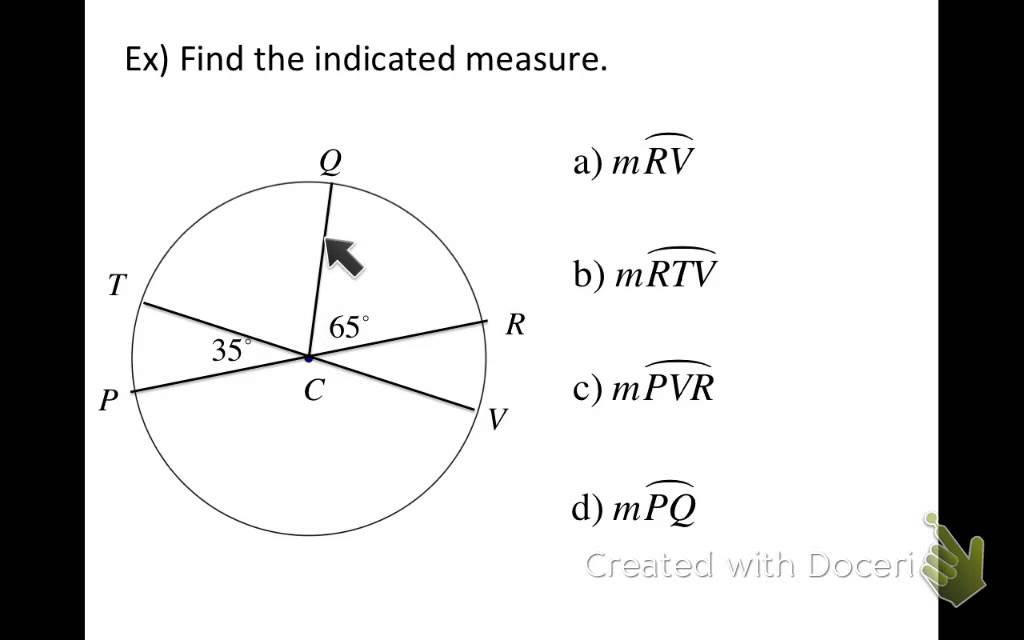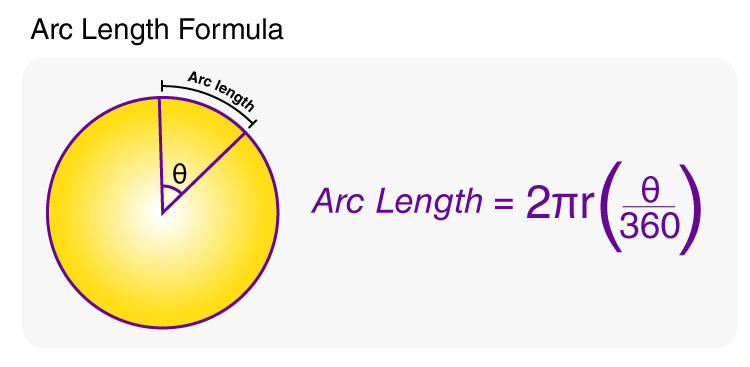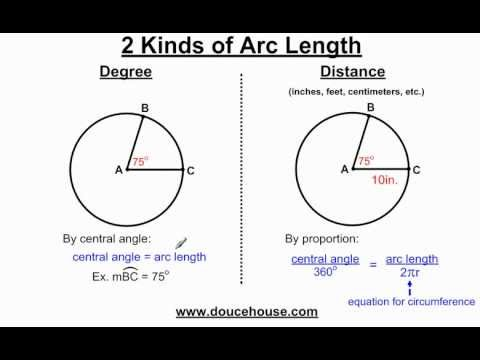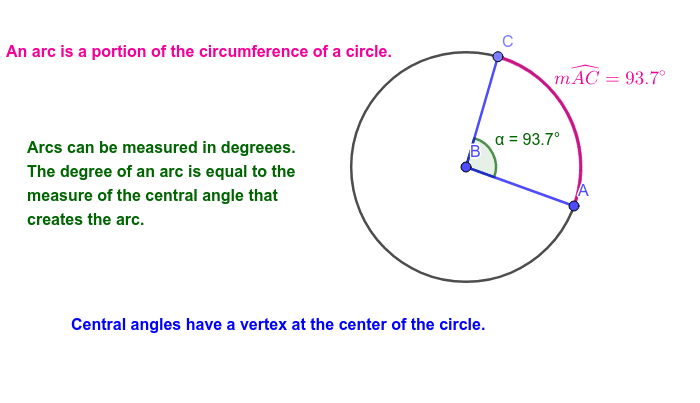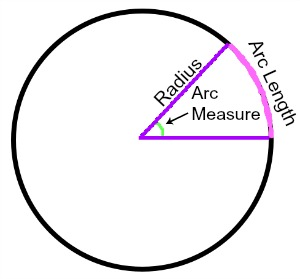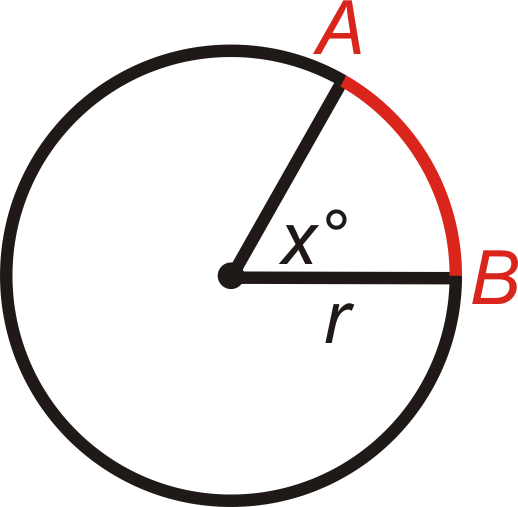# The History of Arc Measurement:

##### The History of Arc Measurement:

Introduction:Arc measurement is a fundamental concept in geometry and trigonometry that involves determining the length of a curved segment of a circle or any other curve. It has applications in various fields, including engineering, architecture, physics, and astronomy. In this article, we will explore the history of arc measurement, highlight its timeline of development, provide answers to frequently asked questions, share interesting facts, and conclude with the significance of arc measurement in modern-day applications.The History of Arc Measurement:The study of arc measurement dates back to ancient civilizations when early mathematicians and astronomers recognized the need to understand and quantify curved shapes.The study of arc measurement dates back to ancient civilizations when early mathematicians and astronomers recognized the need to understand and quantify curved shapes.

 Q: What are the common units of arc measurement? A: Arc measurement is typically expressed in degrees, minutes, and seconds (° ' '), radians, or as a fraction of the circumference of the circle. Q: How is arc length calculated? A: The length of an arc can be calculated using various formulas, depending on the given information, such as the central angle or the radius of the circle. Q: What are some practical applications of arc measurement? A: Arc measurement is essential in fields such as architecture for designing curved structures, engineering for constructing roads and bridges with curved alignments, and astronomy for studying the positions and movements of celestial objects.
##### Conclusion:

Arc measurement has a rich history that spans ancient civilizations to modern times. It has played a vital role in our understanding of curved shapes, trigonometry, and various scientific and engineering disciplines. The contributions of ancient mathematicians and astronomers, as well as the ongoing advancements in mathematics and technology, continue to shape our ability to accurately measure and comprehend arcs. Today, arc measurement remains an essential tool in countless applications, enabling us to analyze and work with curves in fields ranging from architecture and engineering to astronomy and physics.

##### Timeline of Arc Measurement:
 Ancient Egypt (2700 BCE): The Rhind Mathematical Papyrus contains references to the calculation of the areas of circular fields, which involve understanding arcs. Ancient Greece (6th century BCE): Mathematicians like Thales and Pythagoras developed theorems related to circles and their arcs. Archimedes (3rd century BCE): Archimedes made significant contributions to arc measurement, including calculating the area of a sector of a circle. Ptolemy (2nd century CE): Ptolemy introduced the concept of chord tables, which facilitated the calculation of arc lengths using trigonometric functions. Middle Ages (9th-14th centuries): Arab mathematicians, such as Al-Khwarizmi and Al-Biruni, further advanced arc measurement and trigonometry, introducing concepts like sine and tangent. Renaissance (15th-16th centuries): Mathematicians like Leonardo da Vinci and Johannes Kepler made breakthroughs in understanding and calculating arcs, laying the foundation for modern trigonometry.
##### Interesting Facts about Arc Measurement:
 The word 'arc' comes from the Latin word 'arcus,' meaning bow or curve. Ancient civilizations, such as the Babylonians and Mayans, developed their own methods of arc measurement. The radian, a unit of measurement for angles, is defined as the angle subtended by an arc with a length equal to the radius of the circle.
##### Image Gallery: